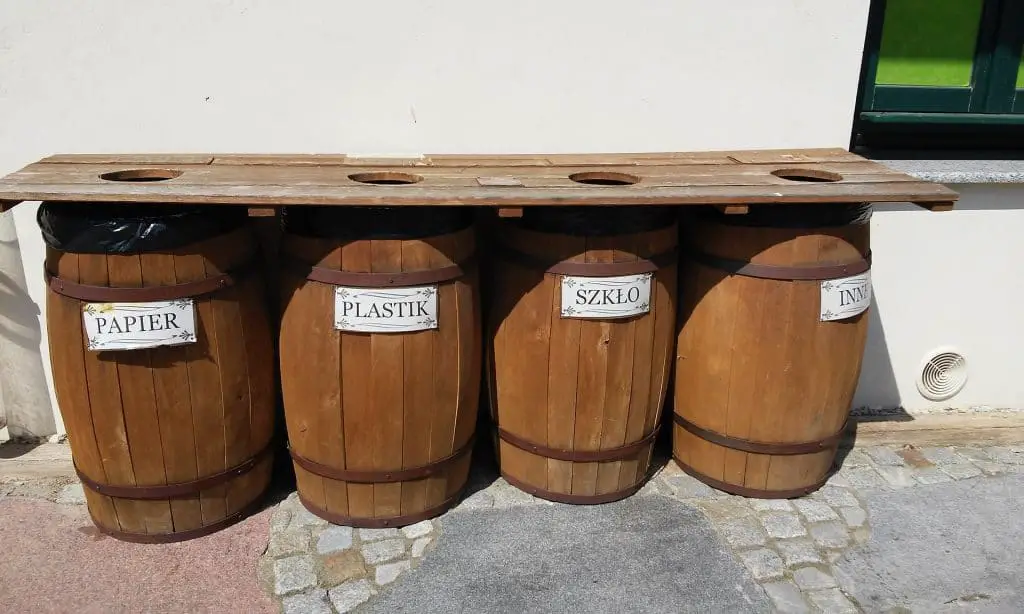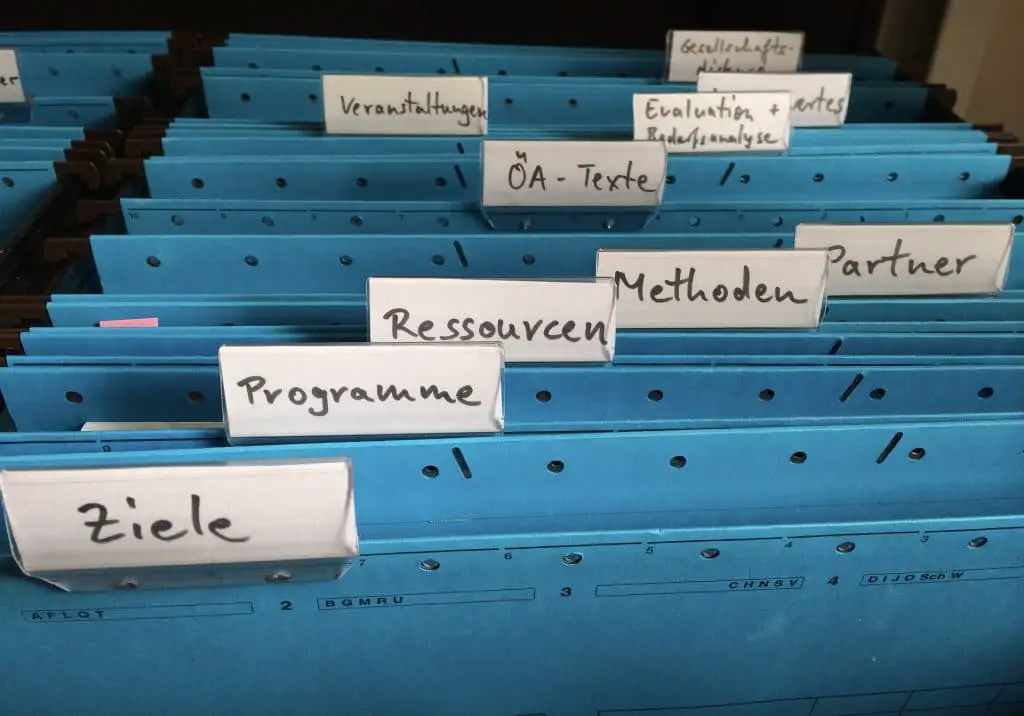">

### What Is A Merge Sort Algorithm And How It Is UsedA primary function of every computer system is to organize data for effective use in analysis, reporting, or presentation purposes. You certainly cannot expect to logically make sense of data that is presented in a random sequence, and make judgements or decisions based on the information.

To solve that problem, computer programmers and mathematicians have created a variety of sorting algorithms that transform non-sequenced data into elements that are sorted into sets of records that provide information in a meaningful manner for business or scientific use.

In today’s sophisticated computer systems that commonly utilize extremely high data volumes for intelligent analysis – referred to as “big data”, efficient sorting techniques are more critical than ever before.

A merge sort algorithm is one of the more commonly-used and powerful solutions for sorting data structures and data content quickly and efficiently.

## Sorting Efficiently with a Merge Sort AlgorithmThere are many options available to computer application developers for sorting sets of data to generate organized output.

Selection of the algorithm to be utilized is to some extent dependent on the language being utilized (ex: C++ “sort()” function can select a different algorithm depending on the array presented for sorting. Its native algorithm is the Introsort function, a blend of heapsort, insertion sort, and quicksort methodology. Depending on the depth of recursion of the array, quicksort or heap sort may be performed. For extremely small arrays, the high performance of insertion sort algorithm will be selected.

When executing a sort function in Python, a combination of insertion sort and merge sort will be used, known as Timsort.

A merge sort algorithm will sort an array into the desired sequence quickly and efficiently, utilizing a divide and conquer methodology. As with many sort algorithms, with a merge sort algorithm the array test will detect the size of the array, and if the size is 0 or 1, consider the data sorted with no processing required.

## What is a Divide and Conquer Algorithm?

But what is a merge sort algorithm, and what makes it different from other sorting techniques?

Merge sort is just one of several divide and conquer algorithms that accomplishes its functions in a multi-step process:

• Divide the array intotwo equal smaller arrays for processing efficiently

This is a simple process of dividing the array size by 2 to determine the midpoint, and creating the two subsets

• Solve the sequencing of each subarray individually – conquer the problem

This is also a straight-forward process involving recursive calls for each subarray to execute the sort process

• Combine the sorted subarrays back into the complete original array, now in sequence – this is the merge function that gives the algorithm its name, and requires heavy comparison processing to create the final result set

This divide, conquer, combine process can be performed much more efficiently than other methods such as insertion sort algorithm, which can take a considerable amount of processing time when arrays exceed more than minimal depth.

Array depth is one of the most important elements in determining the sort algorithm that will perform most efficiently when implemented in your solution. Other considerations include space requirements, memory available, and overall performance.

Since a merge sort algorithm will generate additional arrays in memory while processing the input set (divide), space is an important consideration in using merge sort for large arrays. Your trade-off is in performance – time complexity is a major advantage in using a divide and conquer algorithm like merge sort. Since these algorithms create subarrays as part of their basic functions, they are recursive in execution.

Factors for consideration in sort algorithm selection are available on websites for your comparison and decision-making purposes.

## Merge Sort VariationsThere are multiple variations or implementations of merge sort algorithms, providing options and flexibility in your choice of sorting methodology:

### 3-way merge sort

In a 3-way merge sort, instead of sub-setting the input array into two subarrays, three equally-sized separate arrays are created, sorted, then merged. Although the time complexity would seem to be reduced due to the smaller arrays being sorted, the increased number of comparisons required in the merge operation will raise the time complexity during that phase.

### Bottom-up Implementation

Bottom-up processing utilizes indices and two buffers to iteratively merge sub-lists between the buffers to sort elements into the sorted array. The result is a non-recursive merge sort, contrary to the typical recursive nature of other merge sort variations.

### Polyphase Merge Sort

This variation of a bottom-up merge sort is geared for external data sources where multiple files are being sorted, often including data stored on a hard drive or even a tape device. This includes data sets that will be uneven or unknown in their array sizes, being external input to the algorithm. Due to that criteria, polyphase merge sorts are not stable in nature.

### Natural Merge

Similar to the processing of a bottom-up merge, natural merge further examines any existing sorted elements (naturally-occurring sequenced data), and takes advantage to move these elements in a single pass to the result set. In a perfect case, the array will be found to be in sequence, resulting in a single pass to create the solution. Even in a partially-sequenced array, the impact can be improved performance though fewer passes to solve the problem.

### Oscillating Merge

Do you ever deal with data from tape drives, especially those that can read backwards? Oscillating merge sort algorithm was designed with that technology in mind. This variation of merge sort intersperses the input data with the merge process, rather than reading the entire set of data before merging can begin.

## Pros and Cons of a Merge Sort Algorithm

Not all sort algorithms are created equal – in fact, there are significant differences that will impact your decision on the best sort algorithm for solving your problem.

### Pros

• Merge sort utilizes additional space over the original array to create its subsets of data to solve the problem and create sorted output.
• A merge sort algorithm will process large arrays with reduced time complexity over many other options, notably an insertion sort algorithm.
• Where stability is an important factor for your application, merge sort is a viable choice, since it is a stable algorithm. Stability means that where values being sorted are equal in multiple elements, the resulting output will retain the original sequence of those elements.

### Cons

• Space restrictions –since additional space is required to create the subsets of data for divide and conquer algorithms, you need to have space available to utilize this sort method.
• Small arrays – where very small arrays will be sorted, other non-recursive, single-pass algorithms such as insertion sort may be more efficient.

Since the merge step makes an additional copy of the array to accomplish its work, extra space is required. While some algorithms such as insertion sort and selection sort do their work “in place” and are therefore preferred where space is at a premium, merge sort is not an in-place algorithm.

There is an exception to the requirement for a merge sort algorithm’s need for additional space to process – use of a linked list. Due to the nature of how linked lists reside in memory, no additional space is required for a merge sort with linked lists.

## Factors for Choosing the Best Sort AlgorithmNow that you’re comfortable with the concept of what a merge sort algorithm is, your dilemma may be what sort algorithm to utilize in your application. Big O Notation is a representation of how algorithms will perform, based on primary factors:

• Time Complexity
• Space Complexity
• Array Size

Considering those factors plus any special requirements you have in your problem (such as stability issues mentioned earlier), you can make the decision on the algorithm that will perform best for your data and meet your application performance goals.

Where space is not a major consideration, there are additional sort algorithms to be explored for potentially improving your application performance and efficiency:

• QuickSort
• Heap Sort
• Bucket Sort
• Bubble Sort

There are additional sort algorithms available for your applications, each with their own pros and cons. Some are more useful when used with certain programming languages or may be more useful for website applications (such as insertion sort algorithms).

Utilizing sort selection tools and Big O Notation guidelines can help you determine the best sort algorithm for your implementation.

Keywords:What is a merge sort algorithm, merge sort